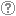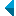# Iskanje po repozitorijuIskalni niz: išči po NaslovAvtorOpisKljučne besedePolno besediloLeto izida INALIIN NE išči po NaslovAvtorOpisKljučne besedePolno besediloLeto izida INALIIN NE išči po NaslovAvtorOpisKljučne besedePolno besediloLeto izida INALIIN NE išči po NaslovAvtorOpisKljučne besedePolno besediloLeto izida Vrsta gradiva: Vse vrste gradiv Habilitacijsko delo (m4) Višješolska diplomska naloga (m6) Specialistično delo (m3) Diplomsko delo * (dip) Magistrsko delo * (mag) Doktorska disertacija * (dok) Raziskovalni podatki ali korpus (data) * po starem in bolonjskem študiju Jezik: Vsi jezikiSlovenski jezikAngleški jezikNemški jezikHrvaški jezikSrbski jezikBolgarski jezikBosanski jezikČeški jezikFinski jezikFrancoski jezikItalijanski jezikJaponski jezikLitvanski jezikMadžarski jezikNemški jezik (Avstrija)Norveški jezikPoljski jezikRuski jezikSlovaški jezikSrbski jezik (cirilica)Španski jezikŠvedski jezikTurški jezikNeznan jezik Išči po: RUP    FAMNIT - Fakulteta za matematiko, naravoslovje in informacijske tehnologije    FHŠ - Fakulteta za humanistične študije    FM - Fakulteta za management    FTŠ Turistica - Fakulteta za turistične študije - Turistica    FVZ - Fakulteta za vede o zdravju    IAM - Inštitut Andrej Marušič    PEF - Pedagoška fakulteta    UPR - Univerza na Primorskem    ZUP - Založba Univerze na PrimorskemCOBISS    Fakulteta za humanistične študije, Koper    Fakulteta za management Koper in Pedagoška fakulteta Koper    Fakulteta za vede o zdravju, Izola    Knjižnica za tehniko, medicino in naravoslovje, Koper    Turistica, Portorož    Znanstveno-raziskovalno središče Koper Opcije: Prikaži samo zadetke s polnim besedilom Ponastavi

 1 - 8 / 811.Rank-permutable additive mappingsAleksandr Èmilevič Guterman, Anna A. Alieva, Bojan Kuzma, 2006, izvirni znanstveni članekOpis: Let ▫$\sigma$▫ be a fixed non-identical permutation on ▫$k$▫ elements. Additive bijections ▫$T$▫ on the matrix algebra ▫$M_n(\mathbb{F})$▫ over a field ▫$\mathbb{F}$▫ of characteristic zero, with the property that ▫$\rm{rk} (A_1...A_k) = \rm{rk} (A_{\sigma(1)}...A_{\sigma(k)})$▫ implies the same condition on the ▫$T$▫ images, are characterized. It is also shown that the surjectivity assumption can be relaxed, if this property is preserved in both directions.Najdeno v: ključnih besedahPovzetek najdenega: ...▫$k$▫ elements. Additive bijections ▫$T$▫ on the matrix algebra ▫$M_n(\mathbb{F})$▫ over a field ▫$\mathbb{F}$▫ of...Ključne besede: mathematics, linearna algebra, matrix algebra, rank, permutation, additive preserversObjavljeno: 15.10.2013; Ogledov: 2631; Prenosov: 85Polno besedilo (0,00 KB) 2.Q-polynomial distance-regular graphs with a [sub] 1 [equal] 0 and a [sub] 2 [not equal] 0Štefko Miklavič, 2008, izvirni znanstveni članekOpis: Let ▫$\Gamma$▫ denote a ▫$Q$▫-polynomial distance-regular graph with diameter ▫$D \ge 3$▫ and intersection numbers ▫$a_1=0$▫, ▫$a_2 \ne 0$▫. Let ▫$X$▫ denote the vertex set of ▫$\Gamma$▫ and let ▫$A \in {\mathrm{Mat}}_X ({\mathbb{C}})$▫ denote the adjacency matrix of ▫$\Gamma$▫. Fix ▫$x \in X$▫ and let denote $A^\ast \in {\mathrm{Mat}}_X ({\mathbb{C}})$ the corresponding dual adjacency matrix. Let ▫$T$▫ denote the subalgebra of ▫$A{\mathrm{Mat}}_X ({\mathbb{C}})$▫ generated by ▫$A$▫, ▫$A^\ast$▫. We call ▫$T$▫ the Terwilliger algebra of ▫$\Gamma$▫ with respect to ▫$x$▫. We show that up to isomorphism there exists a unique irreducible ▫$T$▫-module ▫$W$▫ with endpoint 1. We show that ▫$W$▫ has dimension ▫$2D-2$▫. We display a basis for ▫$W$▫ which consists of eigenvectors for ▫$A^\ast$▫. We display the action of ▫$A$▫ on this basis. We show that ▫$W$▫ appears in the standard module of ▫$\Gamma$▫ with multiplicity ▫$k-1$▫, where ▫$k$▫ is the valency of ▫$\Gamma$▫.Najdeno v: ključnih besedahPovzetek najdenega: ...▫$A \in {\mathrm{Mat}}_X ({\mathbb{C}})$▫ denote the adjacency matrix of ▫$\Gamma$▫. Fix ▫$x \in X$▫ and... ...dual adjacency matrix. Let ▫$T$▫ denote the sub algebra of ▫$A{\mathrm{Mat}}_X ({\mathbb{C}})$▫ generated by ▫$A$▫, ▫$A^\ast$▫....Ključne besede: mathematics, graph theory, adjacency matrix, distance-regular graph, Terwilliger algebraObjavljeno: 15.10.2013; Ogledov: 3250; Prenosov: 27Polno besedilo (0,00 KB) 3.Jordan [tau]-derivations of locally matrix ringsAjda Fošner, Chen-Lian Chuang, Tsiu Kwen Lee, 2013, izvirni znanstveni članekOpis: Let ▫$R$▫ be a prime, locally matrix ring of characteristic not 2 and let ▫$Q_{ms}(R)$▫ be the maximal symmetric ring of quotients of ▫$R$▫. Suppose that ▫$\delta \colon R \to Q_{ms}(R)$▫ is a Jordan ▫$\tau$▫-derivation, where ▫$\tau$▫ is an anti-automorphism of $R$. Then there exists ▫$a \in Q_{ms}(R)$▫ such that ▫$\delta(x) = xa - a\tau(x)$▫ for all ▫$x \in R$▫. Let ▫$X$▫ be a Banach space over the field ▫$\mathbb{F}$▫ of real or complex numbers and let ▫$\mathcal{B}(X)$▫ be the algebra of all bounded linear operators on ▫$X$▫. We prove that ▫$Q_{ms}(\mathcal{B}(X)) = \mathcal{B}(X)$▫, which provides the viewpoint of ring theory for some results concerning derivations on the algebra ▫$\mathcal{B}(X)$▫. In particular, all Jordan ▫$\tau$▫-derivations of ▫$\mathcal{B}(X)$▫ are inner if ▫$\dim_{\mathbb{F}} X>1$▫.Najdeno v: ključnih besedahPovzetek najdenega: ...complex numbers and let ▫$\mathcal{B}(X)$▫ be the algebra of all bounded linear operators on ▫$X$▫....Ključne besede: mathematics, algebra, anti-automorphism, locally matrix ring, prime ring, Jordan homomorphism, Jordan ▫$\tau$▫-derivation, Banach spaceObjavljeno: 15.10.2013; Ogledov: 2839; Prenosov: 76Polno besedilo (0,00 KB) 4.Jordan triple product homomorphismsBojan Kuzma, 2006, izvirni znanstveni članekOpis: Nondegenerate mappings that preserve Jordan triple product on ▫${\mathscr{M}}_n({\mathbb{F}}$▫ are characterized. Here, ▫$n \ge 3$▫ and ▫$\mathbb{F}$▫ is an arbitrary field.Najdeno v: ključnih besedahPovzetek najdenega: ...mathematics, linear algebra, matrix algebra, Jordan triple product, nonlinear preserver...Ključne besede: mathematics, linear algebra, matrix algebra, Jordan triple product, nonlinear preserverObjavljeno: 15.10.2013; Ogledov: 3236; Prenosov: 134Polno besedilo (0,00 KB) 5.Mappings that preserve pairs of operators with zero triple Jordan productGorazd Lešnjak, Bojan Kuzma, Mirko Dobovišek, Chi-Kwong Li, Tatjana Petek, 2007, izvirni znanstveni članekOpis: Let ▫$\mathbb{F}$▫ be a field and ▫$n \ge 3$▫. Suppose ▫${\mathfrak{G_1,G_2}} \subseteq M_n(\mathbb{F})▫$ contain all rank-one idempotents. The structure of surjections ▫$\phi : \mathfrak{G_1} \to \mathfrak{G_2}$▫ satisfying ▫$ABA = 0 \iff \phi(A)\phi(B)\phi(A) = 0$▫ is determined. Similar results are also obtained for (a) subsets of bounded operators acting on a complex or real Banach space, (b) the space of Hermitian matrices acting on ▫$n$▫-dimensional vectors over a skew-field, (c) subsets of self-adjoint bounded linear operators acting on an infinite dimensional complex Hilbert space. It is then illustrated that the results can be applied to characterize mappings ▫$\phi$▫ on matrices or operators such that ▫$F(ABA) = F(\phi(A)\phi(B)\phi(A))▫$ for all ▫$A,B$▫ for functions ▫$F$▫ such as the spectral norm, Schatten ▫$p$▫-norm, numerical radius and numerical range, etc.Najdeno v: ključnih besedahPovzetek najdenega: ... matrix algebra, Jordan triple product, nonlinear preservers...Ključne besede: matrix algebra, Jordan triple product, nonlinear preserversObjavljeno: 03.04.2017; Ogledov: 1827; Prenosov: 93Polno besedilo (0,00 KB) 6.General preservers of quasi-commutativityGregor Dolinar, Bojan Kuzma, 2010, izvirni znanstveni članekOpis: Let ▫$M_n$▫ be the algebra of all ▫$n \times n$▫ matrices over ▫$\mathbb{C}$▫. We say that ▫$A, B \in M_n$▫ quasi-commute if there exists a nonzero ▫$\xi \in \mathbb{C}$▫ such that ▫$AB = \xi BA$▫. In the paper we classify bijective not necessarily linear maps ▫$\Phi \colon M_n \to M_n$▫ which preserve quasi-commutativity in both directions.Najdeno v: ključnih besedahPovzetek najdenega: ...mathematics, linear algebra, general preserver, matrix algebra, quasi-commutativity...Ključne besede: mathematics, linear algebra, general preserver, matrix algebra, quasi-commutativityObjavljeno: 03.04.2017; Ogledov: 1412; Prenosov: 75Polno besedilo (0,00 KB) 7.On maximal distances in a commuting graphBojan Kuzma, Gregor Dolinar, Polona Oblak, 2012, izvirni znanstveni članekOpis: It is shown that matrices over algebraically closed fields that are farthest apart in the commuting graph must be non-derogatory. Rank-one matrices and diagonalizable matrices are also characterized in terms of the commuting graph.Najdeno v: ključnih besedahPovzetek najdenega: ...mathematics, linear algebra, graph theory, commuting graph, matrix algebra, algebraically closed field, centralizer, distance in...Ključne besede: matematika, linearna algebra, teorija grafov, komutirajoči grafi, matrična algebra, algebraično zaprt obseg, centralizator, razdalja v grafih, mathematics, linear algebra, graph theory, commuting graph, matrix algebra, algebraically closed field, centralizer, distance in graphsObjavljeno: 03.04.2017; Ogledov: 1712; Prenosov: 230Polno besedilo (0,00 KB)Gradivo ima več datotek! Več... 8.Permanent versus determinant over a finite fieldAleksandr Èmilevič Guterman, Gregor Dolinar, Bojan Kuzma, Marko Orel, 2013, objavljeni znanstveni prispevek na konferenciOpis: Let ▫$\mathbb{F}$▫ be a finite field of characteristic different from 2. We study the cardinality of sets of matrices with a given determinant or a given permanent for the set of Hermitian matrices ▫$\mathcal{H}_n(\mathbb{F})$▫ and for the whole matrix space ▫$M_n(\mathbb{F})$▫. It is known that for ▫$n = 2$▫, there are bijective linear maps ▫$\Phi$▫ on ▫$\mathcal{H}_n(\mathbb{F})$▫ and ▫$M_n(\mathbb{F})$▫ satisfying the condition per ▫$A = \det \Phi(A)$▫. As an application of the obtained results, we show that if ▫$n \ge 3$▫, then the situation is completely different and already for ▫$n = 3$▫, there is no pair ofmaps ▫$(\Phi, \phi)$▫, where ▫$\Phi$▫ is an arbitrary bijective map on matrices and ▫$\phi \colon \mathbb{F} \to \mathbb{F}$▫ is an arbitrary map such that per ▫$A = \phi(\det \Phi(A))$▫ for all matrices ▫$A$▫ from the spaces ▫$\mathcal{H}_n(\mathbb{F})$▫ and ▫$M_n(\mathbb{F})$▫, respectively. Moreover, for the space ▫$M_n(\mathbb{F})$▫, we show that such a pair of transformations does not exist also for an arbitrary ▫$n > 3$▫ if the field ▫$\mathbb{F}$▫ contains sufficiently many elements (depending on ▫$n$▫). Our results are illustrated by a number of examples.Najdeno v: ključnih besedahPovzetek najdenega: ...Hermitian matrices ▫$\mathcal{H}_n(\mathbb{F})$▫ and for the whole matrix space ▫$M_n(\mathbb{F})$▫. It is known that for... ...mathematics, linear algebra, matrix theory, permanent, determinant...Ključne besede: mathematics, linear algebra, matrix theory, permanent, determinantObjavljeno: 03.04.2017; Ogledov: 1536; Prenosov: 85Polno besedilo (0,00 KB)
Iskanje izvedeno v 0 sek.
Na vrh